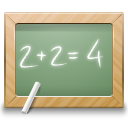Subsections

align_tensor.svdSynopsis

Calculate the singular values and condition number for all alignment tensors.

Defaults

align_tensor.svd(basis_set=`irreducible 5D', tensors=None, precision=4)

Keyword arguments

basis_set: The basis set to operate with.

tensors: A list of the tensors to apply the calculation to. If None, all tensors are used.

precision: The precision of the printed out singular values and condition numbers. The number corresponds to the number of figures to print after the decimal point.

Description

This will perform a singular value decomposition for all alignment tensors and calculate the condition number. The singular values and condition number are dependent on the basis set - linear maps produce identical results whereas non-linear maps result in different values. The basis set can be one of:

`irreducible 5D' -
The irreducible spherical tensor 5D basis set {A-2, A-1, A0, A1, A2}. This is a linear map, hence angles, singular values, and condition number are preserved. These are the spherical harmonic decomposition coefficients.
`unitary 9D' -
The unitary 9D basis set {Sxx, Sxy, Sxz, Syx, Syy, Syz, Szx, Szy, Szz}. This is a linear map, hence angles, singular values, and condition number are preserved.
`unitary 5D' -
The unitary 5D basis set {Sxx, Syy, Sxy, Sxz, Syz}. This is a non-linear map, hence angles, singular values, and condition number are not preserved.
`geometric 5D' -
The geometric 5D basis set {Szz, Sxxyy, Sxy, Sxz, Syz}. This is a non-linear map, hence angles, singular values, and condition number are not preserved. This is also the Pales standard notation.

If the selected basis set is the default of `irreducible 5D', the matrix on which SVD will be performed will be:

| A-2(1) A-1(1) A0(1)  A1(1)  A2(1) |
| A-2(2) A-1(2) A0(2)  A1(2)  A2(2) |
| A-2(3) A-1(3) A0(3)  A1(3)  A2(3) |
|   .      .     .      .      .    |
|   .      .     .      .      .    |
|   .      .     .      .      .    |
| A-2(N) A-1(N) A0(N)  A1(N)  A2(N) |

If the selected basis set is `unitary 9D', the matrix on which SVD will be performed will be:

| Sxx1 Sxy1 Sxz1 Syx1 Syy1 Syz1 Szx1 Szy1 Szz1 |
| Sxx2 Sxy2 Sxz2 Syx2 Syy2 Syz2 Szx2 Szy2 Szz2 |
| Sxx3 Sxy3 Sxz3 Syx3 Syy3 Syz3 Szx3 Szy3 Szz3 |
|  .    .    .    .    .    .    .    .    .   |
|  .    .    .    .    .    .    .    .    .   |
|  .    .    .    .    .    .    .    .    .   |
| SxxN SxyN SxzN SyxN SyyN SyzN SzxN SzyN SzzN |

Otherwise if the selected basis set is `unitary 5D', the matrix for SVD is:

| Sxx1 Syy1 Sxy1 Sxz1 Syz1 |
| Sxx2 Syy2 Sxy2 Sxz2 Syz2 |
| Sxx3 Syy3 Sxy3 Sxz3 Syz3 |
|  .    .    .    .    .   |
|  .    .    .    .    .   |
|  .    .    .    .    .   |
| SxxN SyyN SxyN SxzN SyzN |

Or if the selected basis set is `geometric 5D', the stretching and skewing parameters Szz and Sxx-yy will be used instead and the matrix is:

| Szz1 Sxxyy1 Sxy1 Sxz1 Syz1 |
| Szz2 Sxxyy2 Sxy2 Sxz2 Syz2 |
| Szz3 Sxxyy3 Sxy3 Sxz3 Syz3 |
|  .     .     .    .    .   |
|  .     .     .    .    .   |
|  .     .     .    .    .   |
| SzzN SxxyyN SxyN SxzN SyzN |

For the irreducible spherical tensor basis set, the Am components are defined as

/ 4pi \ 1/2
A0 = | --- |     Szz ,
\  5  /

/ 8pi \ 1/2
A+/-1 = +/- | --- |     (Sxz +/- iSyz) ,
\ 15  /

/ 2pi \ 1/2
A+/-2 = | --- |     (Sxx - Syy +/- 2iSxy) .
\ 15  /

The relationships between the geometric and unitary basis sets are

Szz = - Sxx - Syy,
Sxxyy = Sxx - Syy.

The relax user manual (PDF), created 2020-08-26.